# Covering (of a set)

(diff) ← Older revision | Latest revision (diff) | Newer revision → (diff)

Any family of subsets of the given setwith union.

By a covering of a topological space, a uniform space or, generally, any set having some structure, one understands any covering of this set. However, in the theory of topological spaces it is particularly natural to consider open coverings, i.e. coverings all elements of which are open sets. The considerable importance of open coverings arises because the elements of such a covering contain complete information on the local structure of the space, while the properties of the covering as a whole (in particular, the number of elements, the multiplicity and the combinatorial properties) reflect essential global characteristics of the space.

For example, the Lebesgue dimension dim of a topological space is defined in terms of the language of open coverings: The dimension of a normal spacedoes not exceed a natural numberif in each finite open covering of this space one can inscribe a finite open covering the multiplicity of which at each point (i.e. the number of elements in the covering containing the given point) does not exceed. The relation of one covering being inscribed in another is a basic general elementary relation between coverings. A familyof sets is inscribed in a familyof sets if each element of the familyis contained in some element of the family. The class of paracompact spaces (cf. Paracompact space) is defined in terms of open coverings.

The name subcovering of a coveringof a setis given to any subfamily of the familythat itself is a covering of. The fundamental concepts of compactness, countable and final compactness are defined in terms of subcoverings. A space is compact if in each of its open coverings one can indicate a finite subcovering. A space is countably compact if in each of its countable open coverings one can indicate a finite subcovering. A space is finally compact if in each of its open coverings one can indicate a countable subcovering. Open coverings are used to define abstract combinatorial objects; this has opened up ways of applying algebraic methods in research on topological spaces more general than polyhedra. P.S. Aleksandrov defined the fundamental concept of the nerve of an arbitrary coveringas an abstract complex the vertices of which are put in one-to-one correspondence with the elements ofand where a finite set of these vertices constitutes an abstract simplex if and only if the intersection of the corresponding elements ofis not empty. Systems of open coverings of a space taken together with the relation of being inscribed correspond to systems of abstract complexes related by simplicial mappings, the so-called spectra of complexes.

Closed coverings also play a substantial part in topology; these are coverings in which all elements are closed sets. If all one-point sets in a topological space are closed, an example of a closed covering for this space is provided by the family of all its one-point subsets. However, such a covering does not contain any information on the topology of the space, apart from the fact that the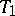-separation axiom applies. Therefore, the requirement of a closed covering should be combined with other substantial restrictions. In particular, it is useful to consider locally finite closed coverings. These are important in dimension theory. Another important example of a closed covering is the covering of a polyhedron by the closed simplices of some complex subdividing this polyhedron.

There are interesting properties of a covering related not to the character of its elements but to the position of them, of which the following are most frequently encountered: the cardinality of a covering (the number of elements in it), local finiteness (for each point in the space there is a neighbourhood that intersects with a finite number of elements from the family of subsets of this space), point finiteness (the set of elements of the covering containing a point taken at random is finite), and star-like finiteness (each element of the covering intersects only with a finite number of elements in the covering).

A family of sets in a topological space is called conservative if the closure of the union of any of its subfamilies is equal to the union of the closures of the elements of that subfamily. Each locally finite family of sets is conservative. Conservative coverings arise in the study of paracompact spaces, where any conservative covering, not merely open an one, is important and not trivial.

An important role is played by the concept of the star of a pointwith respect to a familyof sets (in particular, a covering). It is the union of all elements incontaining, and is usually denoted by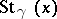. Similarly one defines the star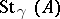of a setwith respect to a family of sets. The concept of a star is used in the fundamental relation of one covering being star-like inscribed in another, which is considerably finer than the relation of being inscribed. A familyof sets is called star-like inscribed in a familyof sets if for each point one can find an element incontaining the star of that point with respect to. The relation of being star-like inscribed for open coverings is important in dimension theory, some criteria for metrization are based on it, and it is one of the basic elementary concepts in defining a uniform structure and a uniform space. It is useful to consider the familyof open coverings of a topological space, directed by the relation of being star-like inscribed in the following sense: For anyandfromthere exists a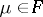which is star-like inscribed both in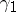and in.

The following characterization of paracompactness in the language of being star-like inscribed is of importance (Morita's theorem): A Hausdorff space is paracompact if and only if one can inscribe in any of its open coverings a star-like open covering.

Star-like inscription does not have so much content for the case of arbitrary (or even closed) coverings. In particular, this is evident from the fact that the family of all one-point subsets of a space is star-like inscribed in any covering of that space.

## Contents

How to Cite This Entry:
Covering (of a set). Encyclopedia of Mathematics. URL: http://encyclopediaofmath.org/index.php?title=Covering_(of_a_set)&oldid=17687
This article was adapted from an original article by A.V. Arkhangel'skii (originator), which appeared in Encyclopedia of Mathematics - ISBN 1402006098. See original article